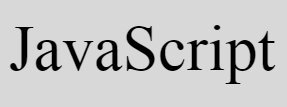# JavaScript Program to Add, Subtract, Multiply, Divide

« Previous Program Next Program »

In this article, you will learn and get code to apply the addition, subtraction, multiplication and division in JavaScript language. There are two programs available here, to perform these four famous mathematical operation in JavaScript:

• Add, Subtract, Multiply, and Divide in JavaScript without user input
• Allow user to enter the numbers using TextBox

## Add, Subtract, Multiply, and Divide in JavaScript

The following JavaScript program find and prints the addition, subtraction, multiplication, and division result of two numbers say 12 and 10 without getting input from user.

This is just a demo program, that how these four mathematical operation performs using JavaScript code. Later on, another program on same task is also given, that receives input from user.

```<!doctype html>
<html>
<body>
<script>
var numOne=12, numTwo=10, res;
res = numOne + numTwo;
document.write("Add = " + res + "<br/>");
res = numOne - numTwo;
document.write("Subtract = " + res + "<br/>");
res = numOne * numTwo;
document.write("Multiply = " + res + "<br/>");
res = numOne/numTwo;
document.write("Divide = " + res + "<br/>");
</script>
</body>
</html>```

Save this code in a file with .html extension. Open the file in a web browser. Here is the output produced by above code:

### Allow User to Enter the Number using TextBox

Now this program allows user to enter the two number and then perform addition, subtraction, multiplication, and division of given two numbers.

```<!doctype html>
<html>
<script>
var numOne, numTwo, res, temp;
function fun()
{
numOne = parseInt(document.getElementById("one").value);
numTwo = parseInt(document.getElementById("two").value);
if(numOne && numTwo)
{
temp = document.getElementById("res");
temp.style.display = "block";
res = numOne + numTwo;
res = numOne - numTwo;
document.getElementById("subtract").value = res;
res = numOne * numTwo;
document.getElementById("multiply").value = res;
res = numOne / numTwo;
document.getElementById("divide").value = res;
}
}
</script>
<body>

<p id="input">Enter First Number: <input id="one">
<br/><br/>
Enter Second Number: <input id="two"></p>
<p id="res" style="display:none;">
Subtraction Result = <input id="subtract"><br/><br/>
Multiplication Result = <input id="multiply"><br/><br/>
Division Result = <input id="divide"></p>

</body>
</html>```

Here is its output:

Now fill up both the boxes with any two numbers say 12 and 10. Now click on the button, Add, Subtract, Multiply, Divide to display the addition, subtraction, multiplication, and division of two entered numbers as shown in the snapshot given below. This is the final output:

The following CSS code:

`style="display:none;"`

hides an HTML element. Because it is included in a paragraph whose id is res. So initially this paragraph will be hidden.

When user clicks on the button, Add, Subtract, Multiply, Divide, then a function named fun() gets called. And all the statements present inside this function gets executed.

The following JavaScript statement:

`numOne = parseInt(document.getElementById("one").value);`

states that, the int (integer) value of an HTML element whose id is one gets initialized to numOne variable. And the following JavaScript statement:

`temp.style.display = "block";`

states that, an HTML element whose id is stored in temp variable gets visible after executing the above JavaScript statement. Here is another JavaScript statement:

`document.getElementById("add").value = res;`

which states that, the value of res gets printed in an HTML element whose id is add.

#### Live Output of Previous Program

Here is the live output produced by previous JavaScript program on add, subtract, multiply, and divide:

Enter First Number:

Enter Second Number:

JavaScript Online Test

« Previous Program Next Program »